# Project Cost Management

7. Jun 2014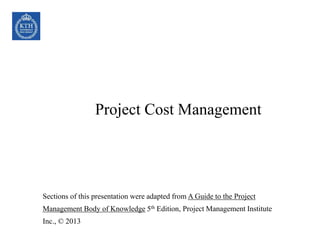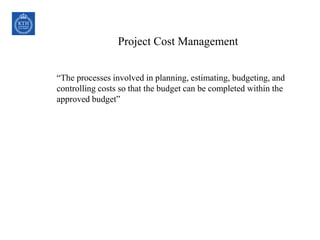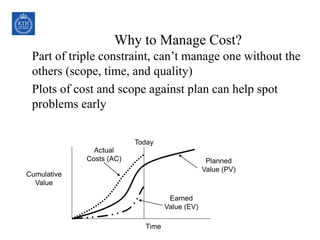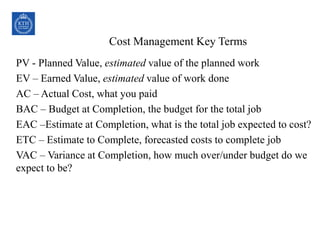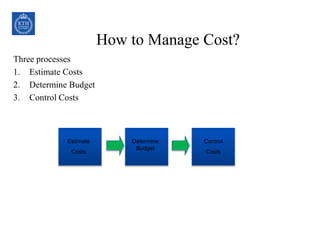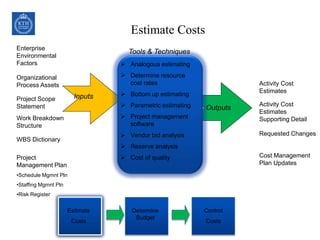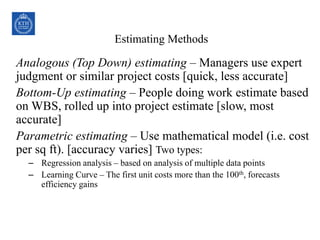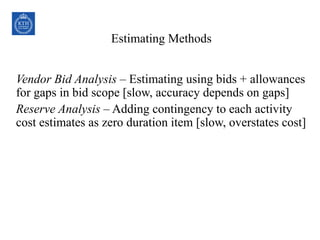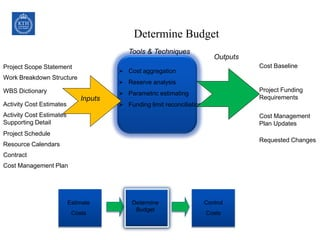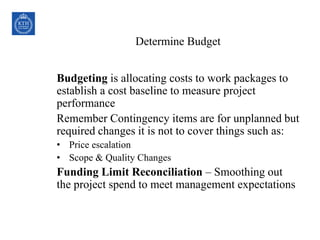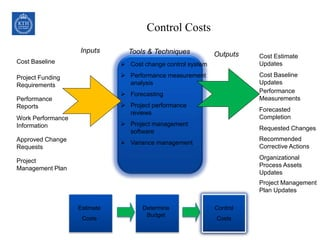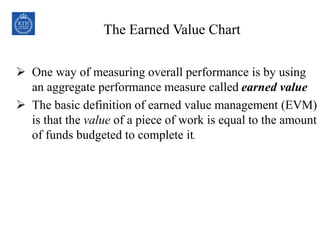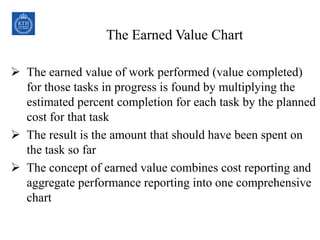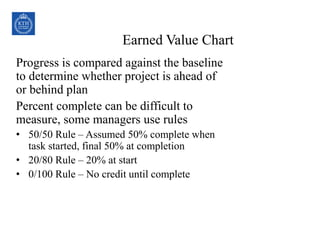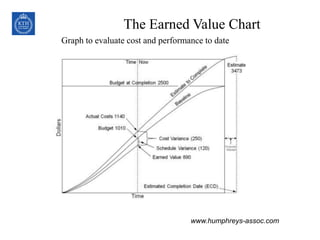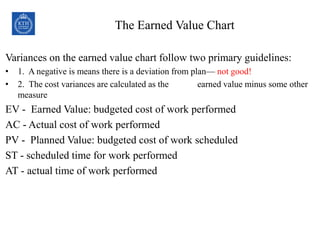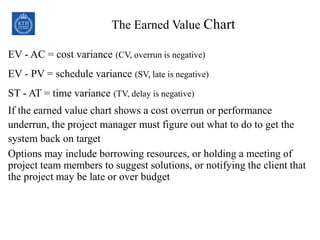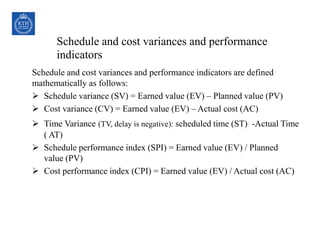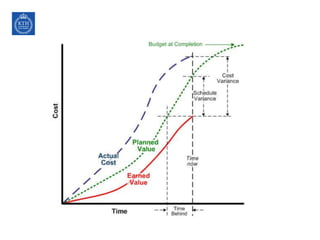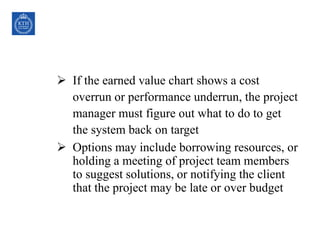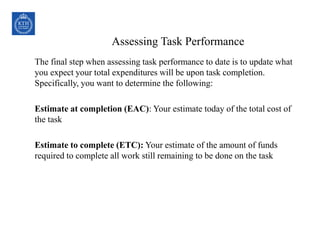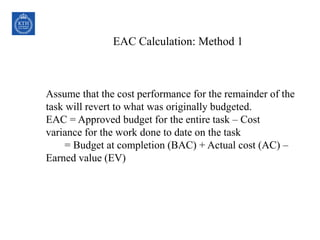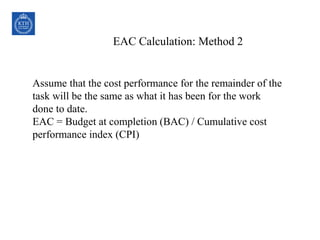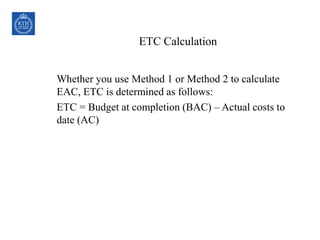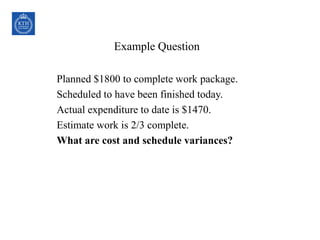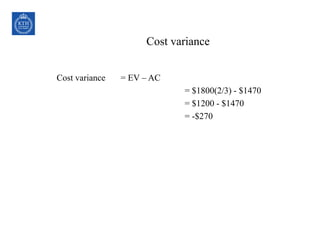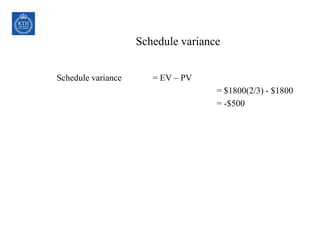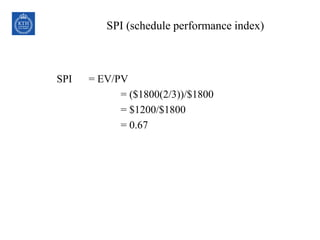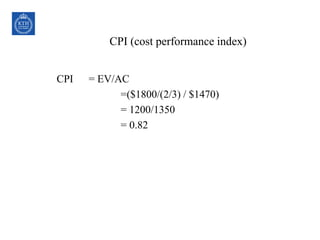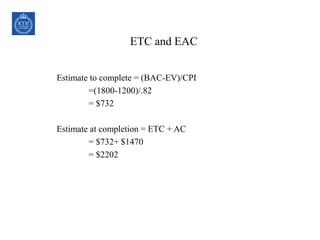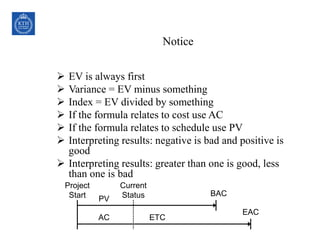1 von 31

### Project Cost Management

• 1. Project Cost Management Sections of this presentation were adapted from A Guide to the Project Management Body of Knowledge 5th Edition, Project Management Institute Inc., © 2013
• 2. Project Cost Management “The processes involved in planning, estimating, budgeting, and controlling costs so that the budget can be completed within the approved budget”
• 3. Why to Manage Cost? Part of triple constraint, can’t manage one without the others (scope, time, and quality) Plots of cost and scope against plan can help spot problems early Cumulative Value Time Planned Value (PV) Actual Costs (AC) Earned Value (EV) Today
• 4. Cost Management Key Terms PV - Planned Value, estimated value of the planned work EV – Earned Value, estimated value of work done AC – Actual Cost, what you paid BAC – Budget at Completion, the budget for the total job EAC –Estimate at Completion, what is the total job expected to cost? ETC – Estimate to Complete, forecasted costs to complete job VAC – Variance at Completion, how much over/under budget do we expect to be?
• 5. How to Manage Cost? Three processes 1. Estimate Costs 2. Determine Budget 3. Control Costs Estimate Costs Determine Budget Control Costs
• 6. Estimate Costs Enterprise Environmental Factors Organizational Process Assets Project Scope Statement  Analogous estimating  Determine resource cost rates  Bottom up estimating  Parametric estimating  Project management software  Vendor bid analysis  Reserve analysis  Cost of quality Inputs Outputs Tools & Techniques Work Breakdown Structure WBS Dictionary Project Management Plan •Schedule Mgmnt Pln •Staffing Mgmnt Pln •Risk Register Activity Cost Estimates Activity Cost Estimates Supporting Detail Requested Changes Cost Management Plan Updates Estimate Costs Determine Budget Control Costs
• 7. Estimating Methods Analogous (Top Down) estimating – Managers use expert judgment or similar project costs [quick, less accurate] Bottom-Up estimating – People doing work estimate based on WBS, rolled up into project estimate [slow, most accurate] Parametric estimating – Use mathematical model (i.e. cost per sq ft). [accuracy varies] Two types: – Regression analysis – based on analysis of multiple data points – Learning Curve – The first unit costs more than the 100th, forecasts efficiency gains
• 8. Estimating Methods Vendor Bid Analysis – Estimating using bids + allowances for gaps in bid scope [slow, accuracy depends on gaps] Reserve Analysis – Adding contingency to each activity cost estimates as zero duration item [slow, overstates cost]
• 9. Determine Budget Project Scope Statement  Cost aggregation  Reserve analysis  Parametric estimating  Funding limit reconciliation Inputs Outputs Tools & Techniques Cost Baseline Project Funding Requirements Cost Management Plan Updates Requested Changes Work Breakdown Structure WBS Dictionary Activity Cost Estimates Activity Cost Estimates Supporting Detail Project Schedule Resource Calendars Contract Cost Management Plan Estimate Costs Determine Budget Control Costs
• 10. Determine Budget Budgeting is allocating costs to work packages to establish a cost baseline to measure project performance Remember Contingency items are for unplanned but required changes it is not to cover things such as: • Price escalation • Scope & Quality Changes Funding Limit Reconciliation – Smoothing out the project spend to meet management expectations
• 11. Control Costs Cost Baseline Project Funding Requirements Performance Reports  Cost change control system  Performance measurement analysis  Forecasting  Project performance reviews  Project management software  Variance management Inputs OutputsTools & Techniques Work Performance Information Approved Change Requests Project Management Plan Cost Estimate Updates Cost Baseline Updates Performance Measurements Forecasted Completion Requested Changes Recommended Corrective Actions Organizational Process Assets Updates Project Management Plan Updates Estimate Costs Determine Budget Control Costs
• 12. The Earned Value Chart  One way of measuring overall performance is by using an aggregate performance measure called earned value  The basic definition of earned value management (EVM) is that the value of a piece of work is equal to the amount of funds budgeted to complete it.
• 13. The Earned Value Chart  The earned value of work performed (value completed) for those tasks in progress is found by multiplying the estimated percent completion for each task by the planned cost for that task  The result is the amount that should have been spent on the task so far  The concept of earned value combines cost reporting and aggregate performance reporting into one comprehensive chart
• 14. Earned Value Chart Progress is compared against the baseline to determine whether project is ahead of or behind plan Percent complete can be difficult to measure, some managers use rules • 50/50 Rule – Assumed 50% complete when task started, final 50% at completion • 20/80 Rule – 20% at start • 0/100 Rule – No credit until complete
• 15. The Earned Value Chart Graph to evaluate cost and performance to date www.humphreys-assoc.com
• 16. The Earned Value Chart Variances on the earned value chart follow two primary guidelines: • 1. A negative is means there is a deviation from plan— not good! • 2. The cost variances are calculated as the earned value minus some other measure EV - Earned Value: budgeted cost of work performed AC - Actual cost of work performed PV - Planned Value: budgeted cost of work scheduled ST - scheduled time for work performed AT - actual time of work performed
• 17. The Earned Value Chart EV - AC = cost variance (CV, overrun is negative) EV - PV = schedule variance (SV, late is negative) ST - AT = time variance (TV, delay is negative) If the earned value chart shows a cost overrun or performance underrun, the project manager must figure out what to do to get the system back on target Options may include borrowing resources, or holding a meeting of project team members to suggest solutions, or notifying the client that the project may be late or over budget
• 18. Schedule and cost variances and performance indicators Schedule and cost variances and performance indicators are defined mathematically as follows:  Schedule variance (SV) = Earned value (EV) – Planned value (PV)  Cost variance (CV) = Earned value (EV) – Actual cost (AC)  Time Variance (TV, delay is negative): scheduled time (ST) -Actual Time ( AT)  Schedule performance index (SPI) = Earned value (EV) / Planned value (PV)  Cost performance index (CPI) = Earned value (EV) / Actual cost (AC)
• 20.  If the earned value chart shows a cost overrun or performance underrun, the project manager must figure out what to do to get the system back on target  Options may include borrowing resources, or holding a meeting of project team members to suggest solutions, or notifying the client that the project may be late or over budget
• 21. Assessing Task Performance The final step when assessing task performance to date is to update what you expect your total expenditures will be upon task completion. Specifically, you want to determine the following: Estimate at completion (EAC): Your estimate today of the total cost of the task Estimate to complete (ETC): Your estimate of the amount of funds required to complete all work still remaining to be done on the task
• 22. EAC Calculation: Method 1 Assume that the cost performance for the remainder of the task will revert to what was originally budgeted. EAC = Approved budget for the entire task – Cost variance for the work done to date on the task = Budget at completion (BAC) + Actual cost (AC) – Earned value (EV)
• 23. EAC Calculation: Method 2 Assume that the cost performance for the remainder of the task will be the same as what it has been for the work done to date. EAC = Budget at completion (BAC) / Cumulative cost performance index (CPI)
• 24. ETC Calculation Whether you use Method 1 or Method 2 to calculate EAC, ETC is determined as follows: ETC = Budget at completion (BAC) – Actual costs to date (AC)
• 25. Example Question Planned \$1800 to complete work package. Scheduled to have been finished today. Actual expenditure to date is \$1470. Estimate work is 2/3 complete. What are cost and schedule variances?
• 26. Cost variance Cost variance = EV – AC = \$1800(2/3) - \$1470 = \$1200 - \$1470 = -\$270
• 27. Schedule variance Schedule variance = EV – PV = \$1800(2/3) - \$1800 = -\$500
• 28. SPI (schedule performance index) SPI = EV/PV = (\$1800(2/3))/\$1800 = \$1200/\$1800 = 0.67
• 29. CPI (cost performance index) CPI = EV/AC =(\$1800/(2/3) / \$1470) = 1200/1350 = 0.82
• 30. ETC and EAC Estimate to complete = (BAC-EV)/CPI =(1800-1200)/.82 = \$732 Estimate at completion = ETC + AC = \$732+ \$1470 = \$2202
• 31. Notice  EV is always first  Variance = EV minus something  Index = EV divided by something  If the formula relates to cost use AC  If the formula relates to schedule use PV  Interpreting results: negative is bad and positive is good  Interpreting results: greater than one is good, less than one is bad PV AC ETC EAC BAC Project Start Current Status